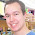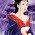## Pages

### Simple Circle in XNA

This post is about creating a simple circle in XNA using VertexPositionColor elements and LineStrip primitive.
Lets call our class Circle.

Members:
`//List of vertices`
`List<VertexPositionColor> _Vertices;`
` `
`//Convert to array to avoid ToArray for every draw`
`VertexPositionColor[] _VertexArray;`
` `
`//Color of the vertices`
`Color _VertexColor;`
` `
`//Main game class`
`Game_Game;`
`BasicEffect _Effect;`
` `
`//Transforms for the circle`
`Matrix _Transforms;`
`Vector3 _Position, _Scale, _Rotation;`
` `
`//Radius of the circle`
`float _Radius;`

Properties:
`public Vector3 Position`
`{`
`get{ return_Position; }`
`set{_Position=value;Transform();}`
`}`
` `
`public Vector3 Rotation`
`{`
`get{return_Rotation;}`
`set{_Rotation=value;Transform();}`
`}`
` `
`public Vector3 Scale`
`{`
`get{return_Scale;}`
`set{_Scale=value;Transform();}`
`}`
` `
`public MatrixTransforms`
`{`
`get{return_Transforms;}`
`set{_Transforms=value;}`
`}`

Constants:
`//This indicates that every 5 degree `
`//a point on the cirumference will be added`
`//Ex: 360/5 = 72 points will be used`
`int DEGREE_INCREMENT = 5;`

Constructor:
`public Circle(Game game, Color color, float radius)`
`{`
`_Game = game;`
`_Effect = new BasicEffect(_Game.GraphicsDevice, null);`
`_Effect.VertexColorEnabled = true;`
`_VertexColor = color;`
`_Transforms = Matrix.Identity;`
`_Radius = radius;`
`_Scale = Vector3.One;`
` `
`_Vertices = new List<VertexPositionColor>();`
`Setup();`
` `
`_VertexArray = _Vertices.ToArray();`
`}`

Setup Function:
`void Setup()`
`{`
`//For each number of points : 360/ DEGREE_INCREMENT`
`for (int i = 0; i <= 360; i+= DEGREE_INCREMENT)`
`{`
`VertexPositionColor tmp = new VertexPositionColor();`
` `
`// X => R * Cos(Angle), Y = 0, Z => R * Sin(Angle)`
`tmp.Position = new Vector3(_Radius * (float)Math.Cos(MathHelper.ToRadians(i)), 0, _Radius * (float)Math.Sin(MathHelper.ToRadians(i)));`
`tmp.Color = _VertexColor;`
`_Vertices.Add(tmp);                `
`} `
`}`

Transform Function:
`//Update transform when pos, rot, scale is changed`
`void Transform()`
`{`
`_Transforms = Matrix.CreateScale(_Scale) * `
`Matrix.CreateFromYawPitchRoll(_Rotation.Y, _Rotation.X, _Rotation.Z) * `
`Matrix.CreateTranslation(_Position);`
`}`

Draw Function:
`public void Draw()`
`{`
`//Tell the device we are sending Vertexpositioncolor elements`
`_Game.GraphicsDevice.VertexDeclaration = new VertexDeclaration(_Game.GraphicsDevice, VertexPositionColor.VertexElements);`
`_Effect.Begin();`
`foreach (EffectPass pass in _Effect.CurrentTechnique.Passes)`
`{`
`pass.Begin();`
` `
`//Set the world, view, projection matrices`
`_Effect.World = _Transforms;`
`_Effect.View = Camera.View;`
`_Effect.Projection = Camera.Projection;`
` `
`//Draw primitive: n points => n-1 lines`
`_Game.GraphicsDevice.DrawUserPrimitives<VertexPositionColor>(PrimitiveType.LineStrip, _VertexArray, 0, _Vertices.Count - 1);`
`pass.End();`
`}`
` `
`_Effect.End(); `
`}`

Screen shots with different Degree increments:

Next up - Sphere

#### 6 comments:

1.Have you unit tested your code??? I hate to say it but I've had a really hard time testing this. I really need a sphere drawing utility to test bounding volumes and ran into a plethora of build errors with your code. Perhaps next time try providing a "complete file" download for people like myself who might be interested.

I would be happy to see if you get it working and choose to post a full file/zip that works. Thanks!

2.@JW: Wow!! Someone actually found my blog! I wrote this for personal memories actually - never thought anyone would read it.
Have no clue where to upload ZIP files in blogger. New to this. Gimme ur email ID - ill mail you the complete solution. May be i missed out some code.

Thanks.
Vik

3.Hi

Do you think u can send me your code for drawing of circle?

I am VERY NEW to game programming and slowly picking up....

4.@Perwyl: How do i contact u?

5.Can you send me the code ?

thanks in advance,

Claudio Lins

6.Sorry Dinho.. it's been too long and I don't have source code.

If u intend on making games then switch over to Unity3D or UDK.
If ur inention is 2 learn gfx programming then I strongly suggest u go with Directx11 (native c++). XNA is way outdated and it's pointless investing ur time on it.

Note: Only a member of this blog may post a comment.